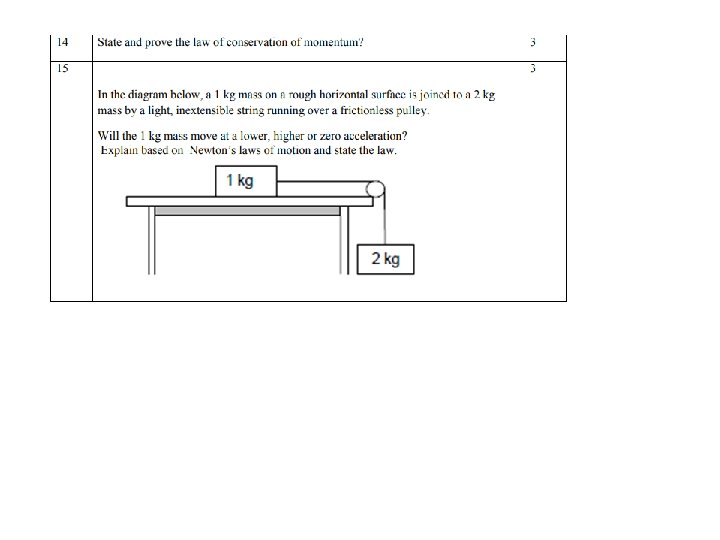# Work Energy and power Work Energy and power

• Slides: 46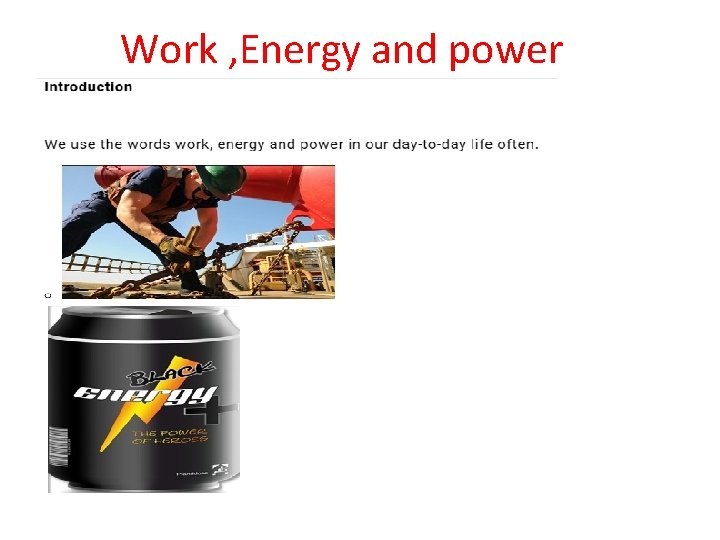Work , Energy and power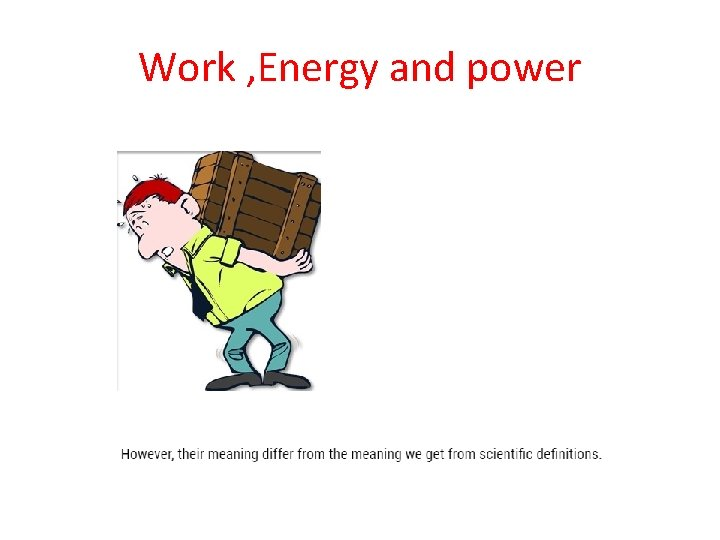Work , Energy and powerScalar product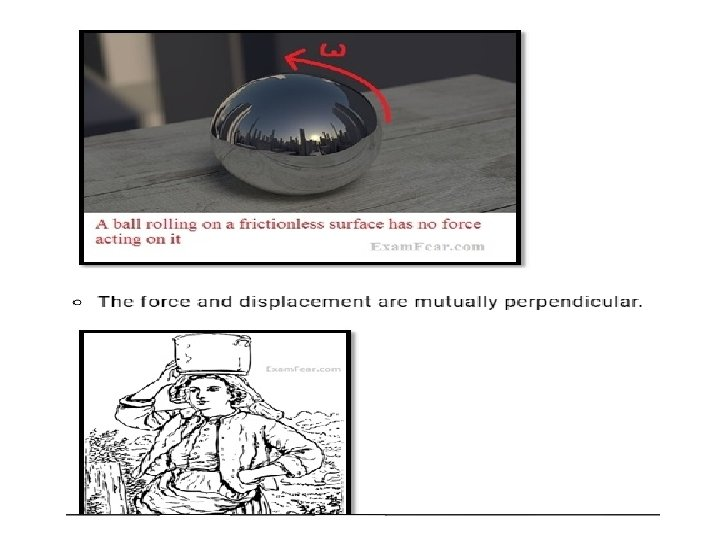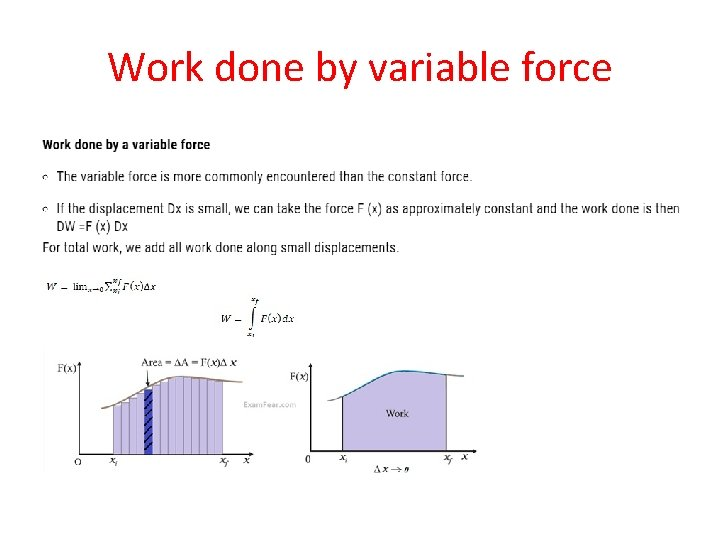Work done by variable force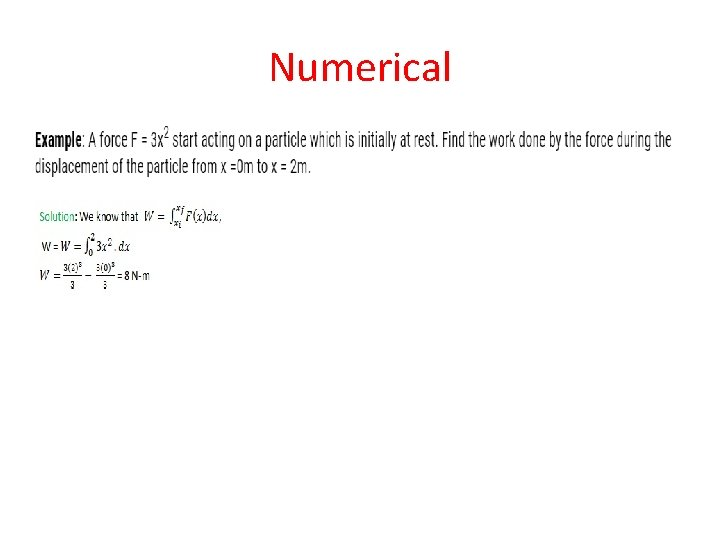NumericalKinetic Energy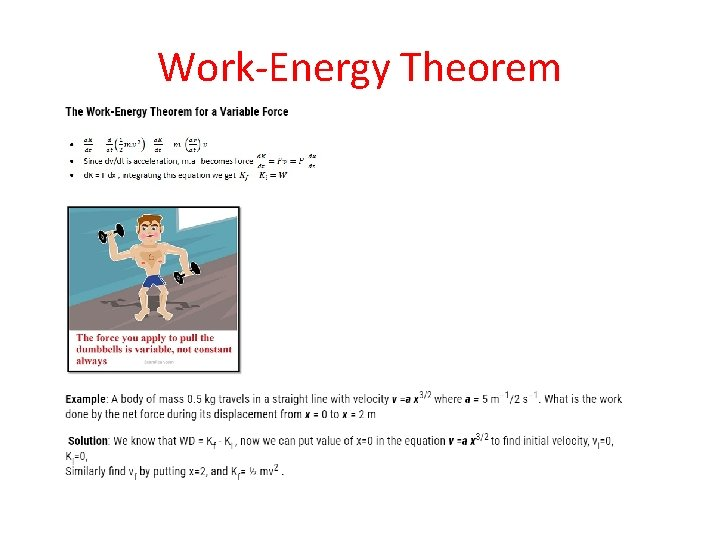Work-Energy TheoremPotential Energy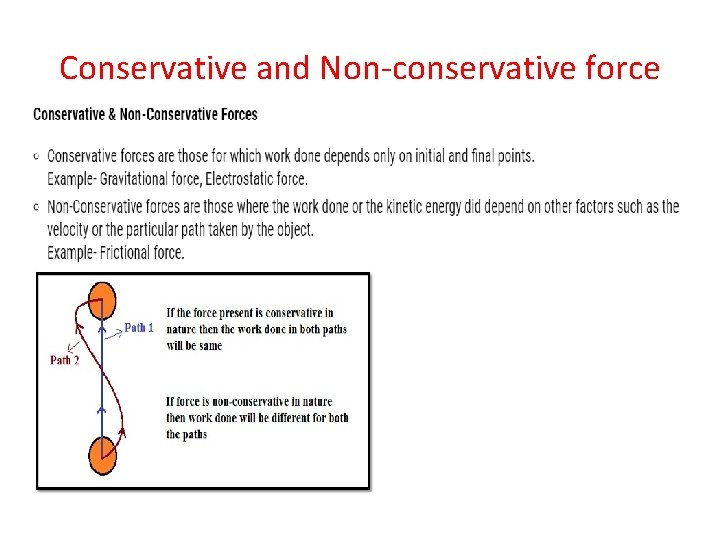Conservative and Non-conservative forceConservation of Mechanical EnergyPotential Energy of a Spring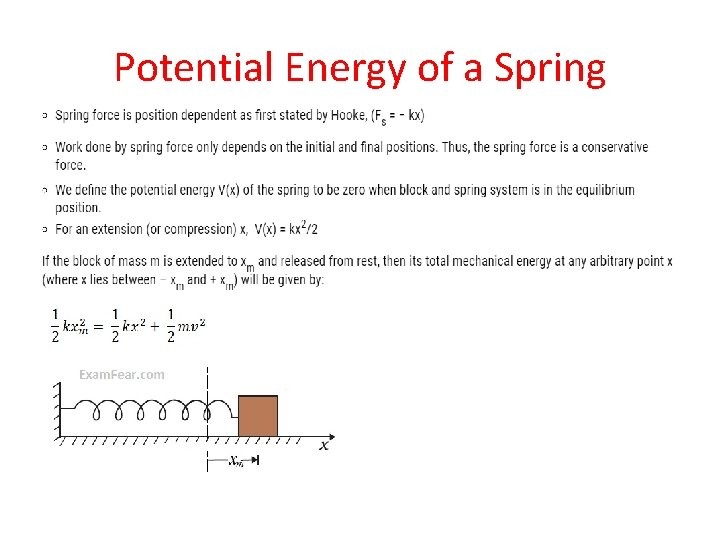Potential Energy of a Spring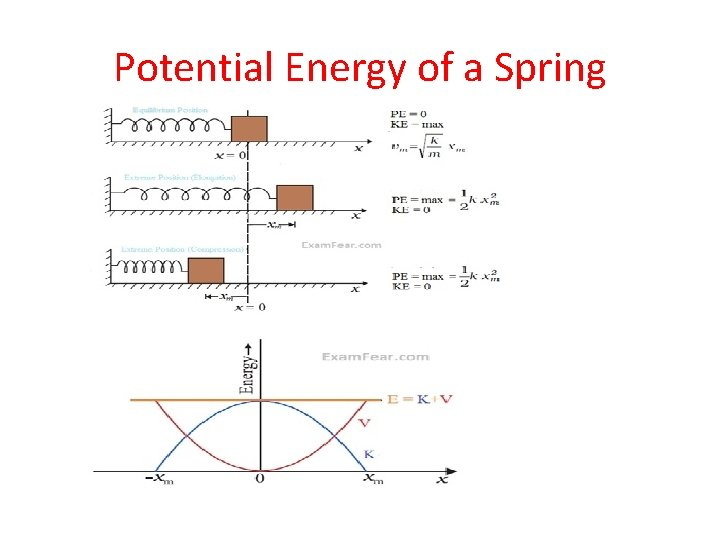Potential Energy of a SpringNumericalVarious Forms of EnergyVarious Forms of Energy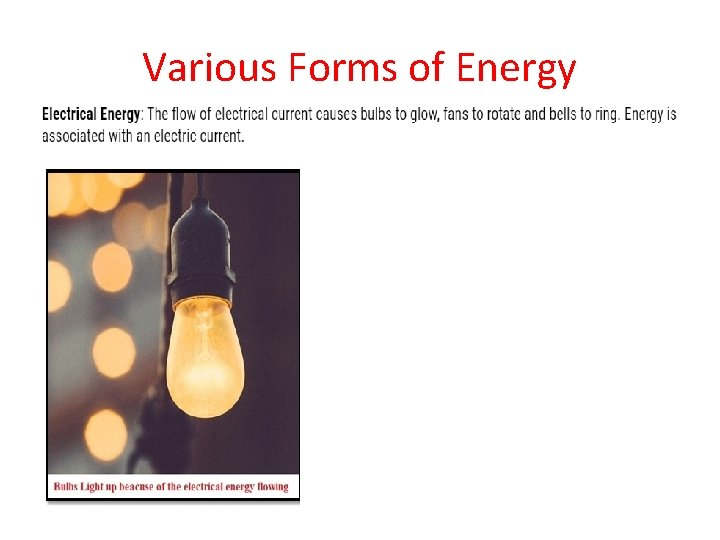Various Forms of Energy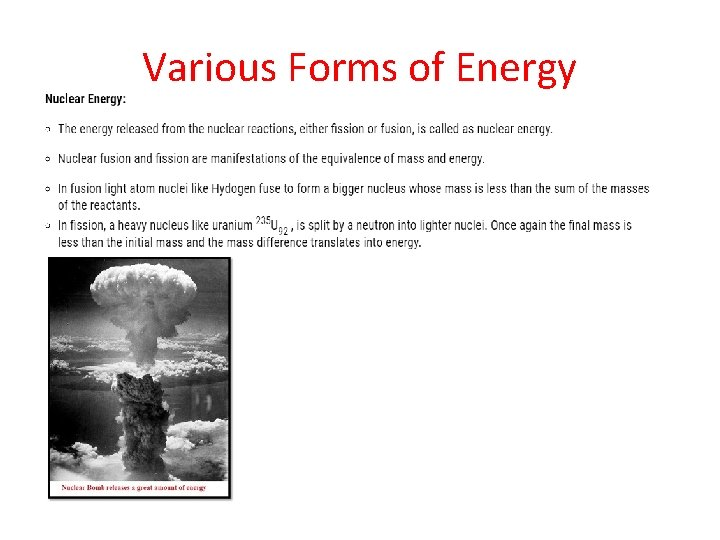Various Forms of Energy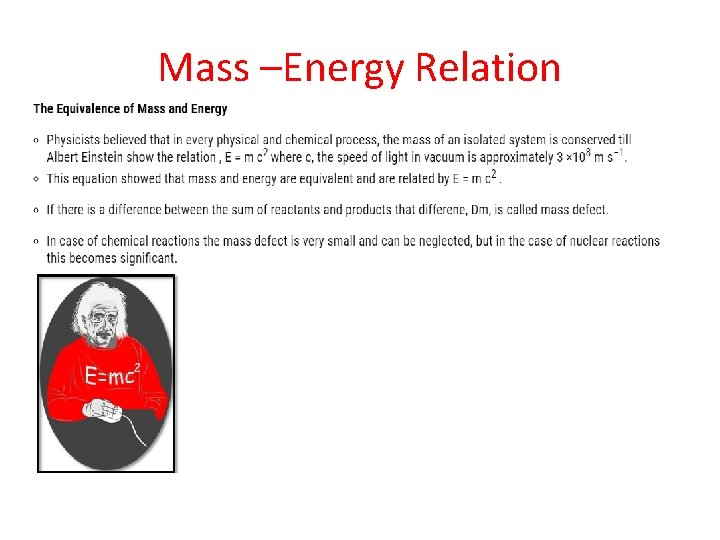Mass –Energy RelationPower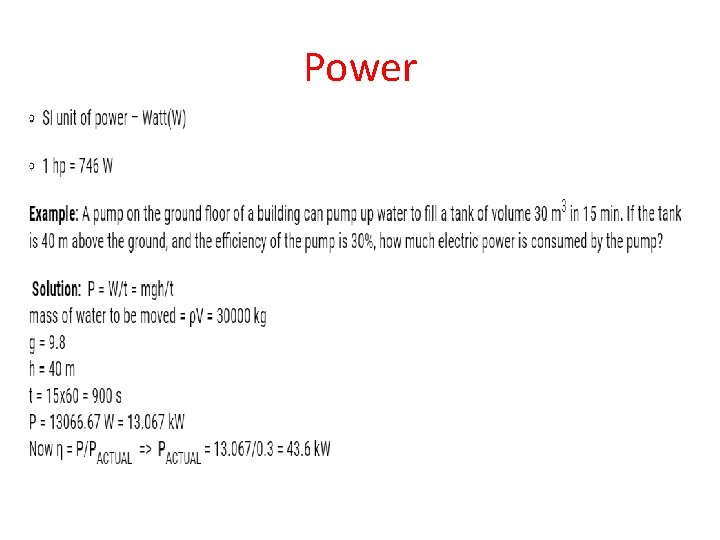PowerCollisionsCollision in one dimension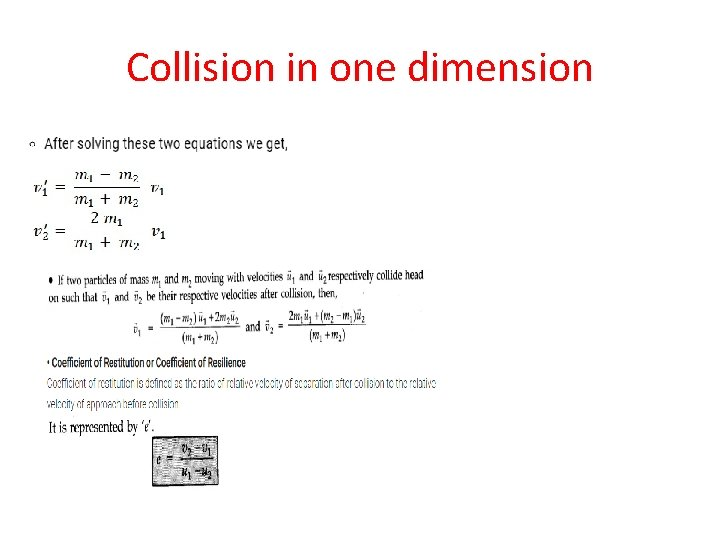Collision in one dimensionCollisions in one dimensions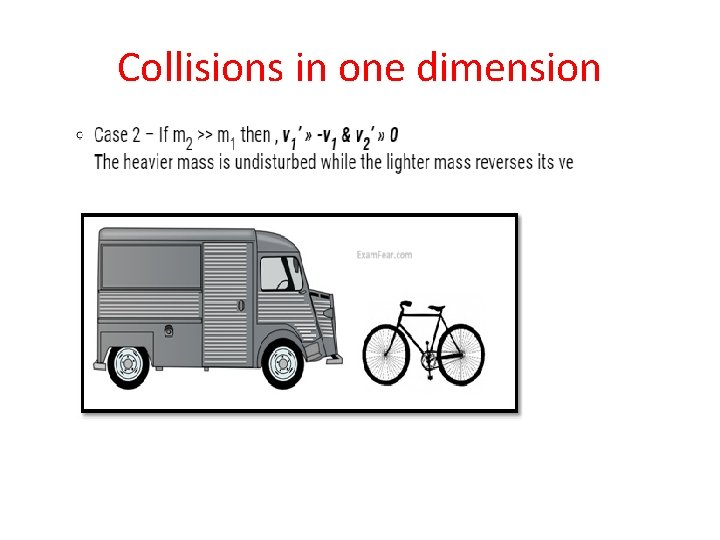Collisions in one dimension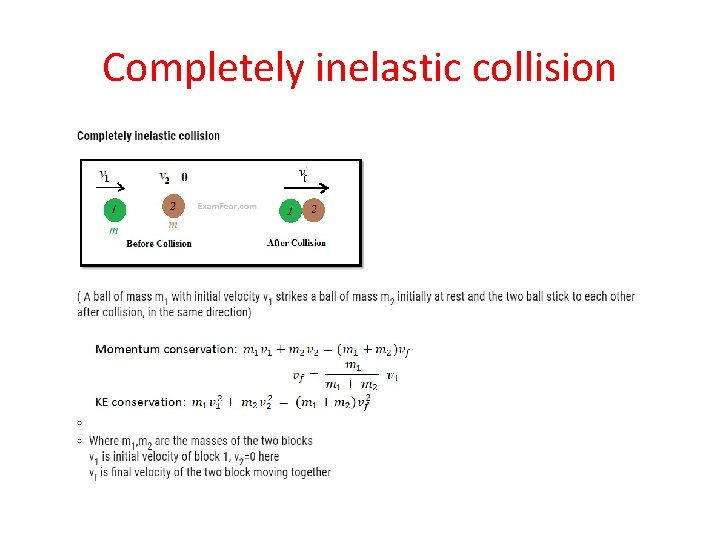Completely inelastic collision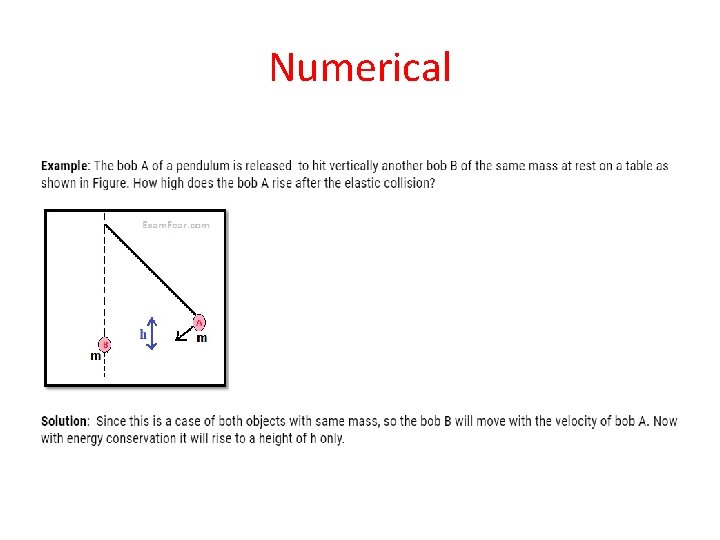Numerical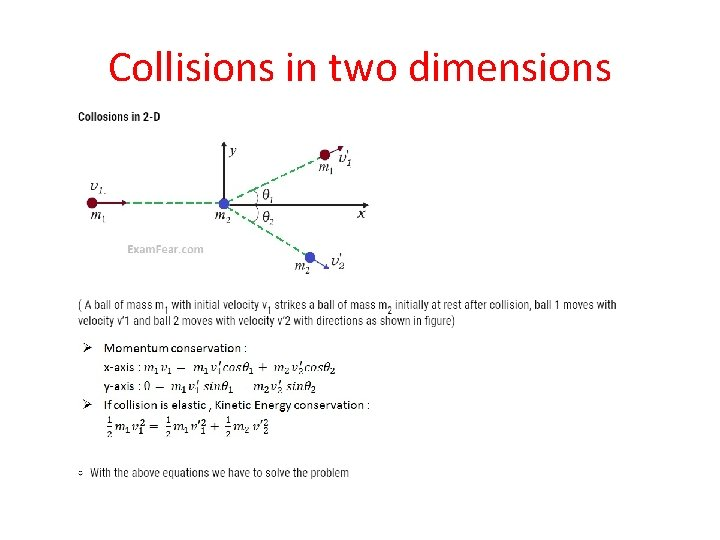Collisions in two dimensions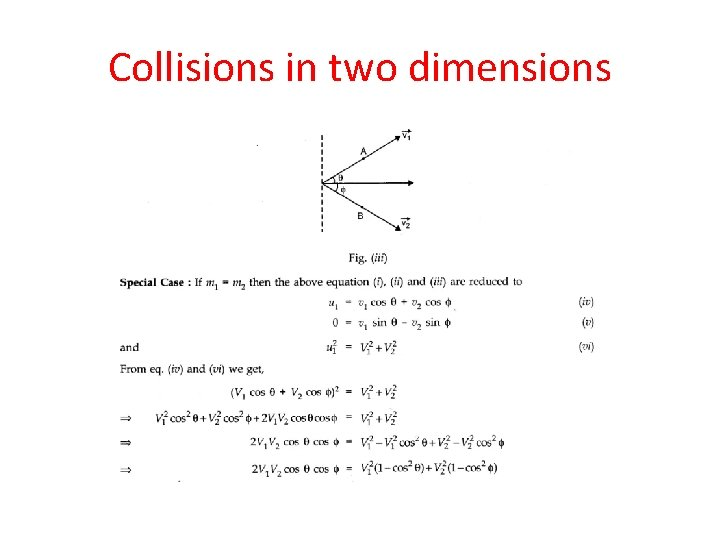Collisions in two dimensions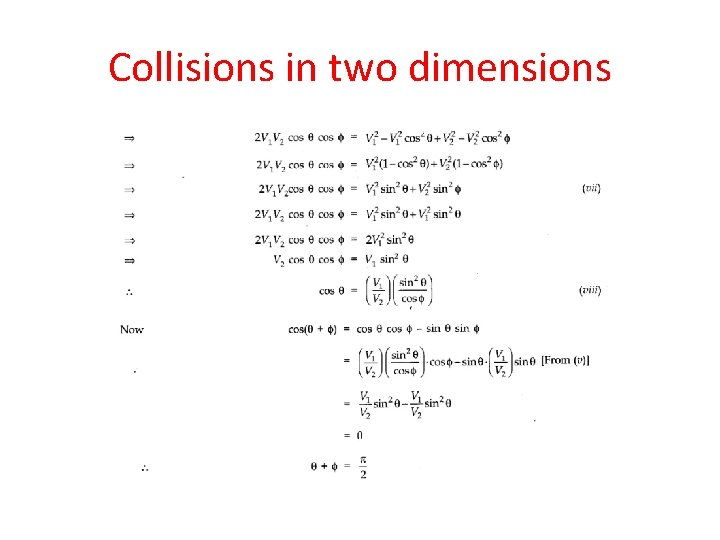Collisions in two dimensions• Example: Two balls initially travelling along the xaxis with opposite velocities collide with each other obliquely, is it possible that they will move in the y-axis after collision? • Solution: Yes, it is possible, if the balls have same mass and travel with same speed. Initially their total momentum in x-direction was zero as their velocities were opposite; also in y-direction it is zero because they do not have velocity in that direction. Now after collision if their velocities are still equal and in opposite direction, the total momentum still remains zero.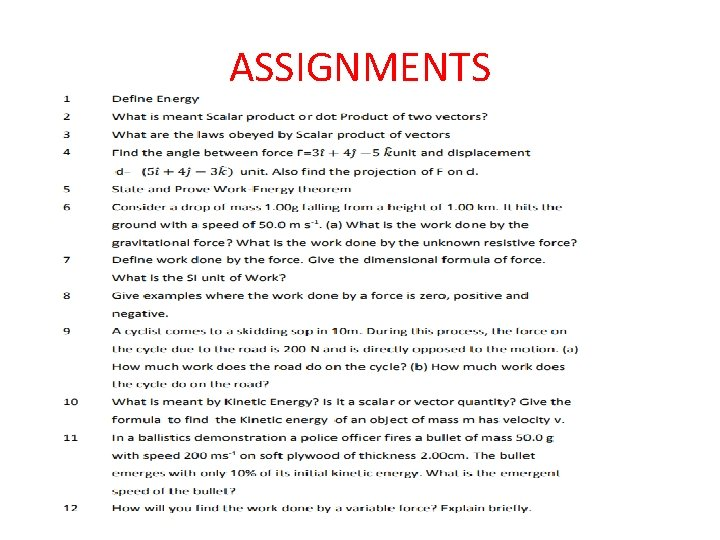ASSIGNMENTS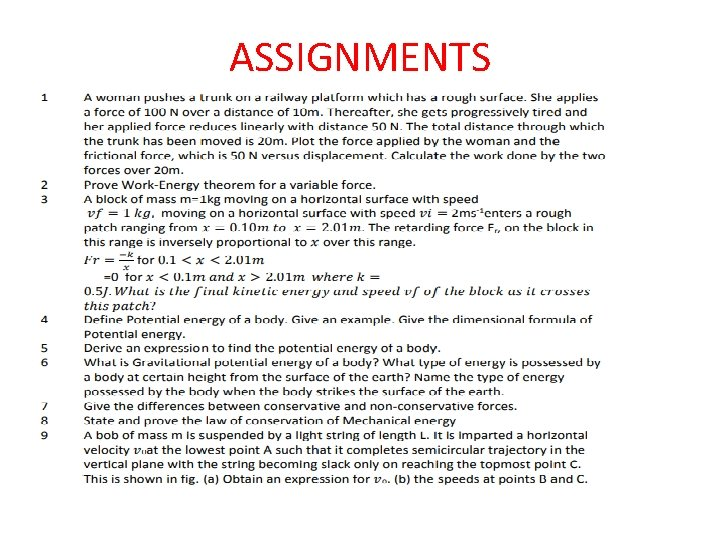ASSIGNMENTSASSIGNMENTS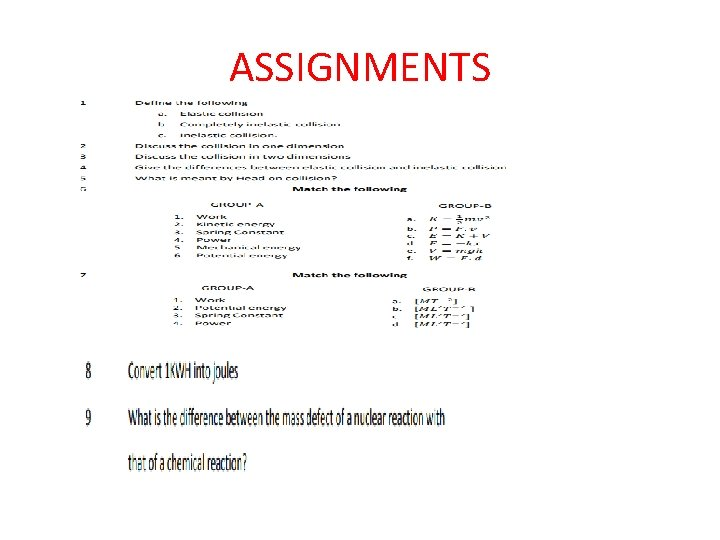ASSIGNMENTSASSIGNMENTSNUMERICAL PROBLEMS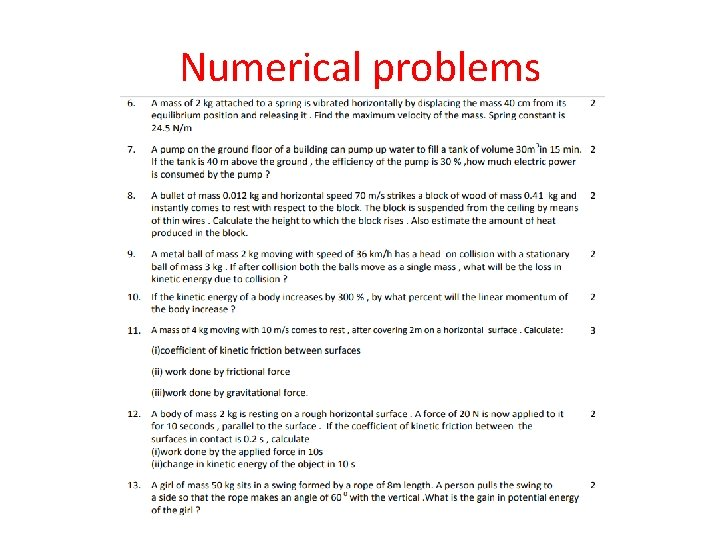Numerical problemsNumerical problemsNumerical problemsNumerical problems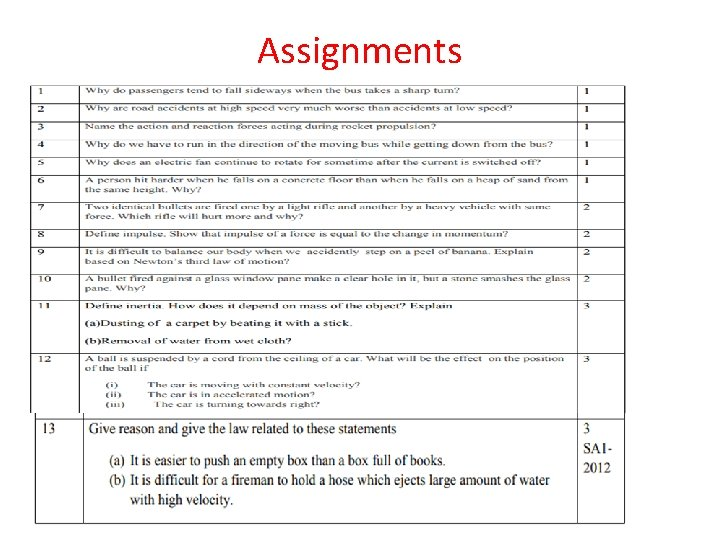Assignments